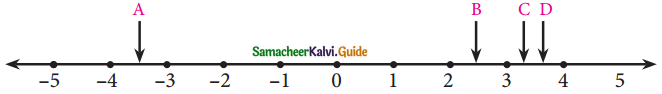Students can download Maths Chapter 2 Real Numbers Ex 2.1 Questions and Answers, Notes, Samacheer Kalvi 9th Maths Guide Pdf helps you to revise the complete Tamilnadu State Board New Syllabus, helps students complete homework assignments and to score high marks in board exams.

## Tamilnadu Samacheer Kalvi 9th Maths Solutions Chapter 2 Real Numbers Ex 2.1

Question 1.
Which arrow best shows the position of $$\frac{11}{3}$$ on the number line?Solution:
D represent $$\frac{11}{3}$$ on the number line.Question 2.
Find any three rational numbers between $$\frac{-7}{11}$$ and $$\frac{2}{11}$$
Solution:
Three rational numbers between $$\frac{-7}{11}$$ and $$\frac{2}{11}$$
$$\frac{-6}{11}$$, $$\frac{-5}{11}$$, $$\frac{-4}{11}$$, ……… $$\frac{1}{11}$$Question 3.
Find any five rational numbers between
(i) $$\frac{1}{4}$$ and $$\frac{1}{5}$$
Solution:
Converting the given rational numbers with the same denominators.
$$\frac{1}{4}$$ = $$\frac{1×30}{4×30}$$ = $$\frac{30}{120}$$
$$\frac{1}{5}$$ = $$\frac{1×24}{5×24}$$ = $$\frac{24}{120}$$
Five rational numbers between $$\frac{30}{120}$$ and $$\frac{24}{120}$$ are $$\frac{25}{120}$$, $$\frac{26}{120}$$, $$\frac{27}{120}$$, $$\frac{28}{120}$$ and $$\frac{29}{120}$$
Five rational numbers between $$\frac{1}{4}$$ and $$\frac{1}{5}$$ are $$\frac{25}{120}$$, $$\frac{26}{120}$$, $$\frac{27}{120}$$, $$\frac{28}{120}$$ and $$\frac{29}{120}$$
Other Method:
A rational numbers between $$\frac{1}{4}$$ and $$\frac{1}{5}$$ = $$\frac{1}{2}$$($$\frac{1}{4}$$+$$\frac{1}{5}$$) = $$\frac{1}{2}$$($$\frac{5+4}{20}$$) = $$\frac{1}{2}$$ × $$\frac{9}{20}$$ = $$\frac{9}{40}$$
A rational numbers between $$\frac{1}{4}$$ and $$\frac{9}{40}$$ = $$\frac{1}{2}$$($$\frac{1}{4}$$+$$\frac{9}{40}$$) = $$\frac{1}{2}$$($$\frac{10+9}{40}$$) = $$\frac{19}{80}$$
A rational numbers between $$\frac{1}{4}$$ and $$\frac{19}{80}$$ = $$\frac{1}{2}$$($$\frac{1}{4}$$+$$\frac{19}{20}$$) = $$\frac{1}{2}$$($$\frac{20+19}{80}$$) = $$\frac{39}{160}$$
A rational numbers between $$\frac{1}{4}$$ and $$\frac{39}{160}$$ = $$\frac{1}{2}$$($$\frac{1}{4}$$+$$\frac{39}{160}$$) = $$\frac{1}{2}$$($$\frac{40+39}{160}$$) = $$\frac{79}{320}$$
A rational numbers between $$\frac{1}{4}$$ and $$\frac{79}{320}$$ = $$\frac{1}{2}$$($$\frac{1}{4}$$+$$\frac{79}{320}$$) = $$\frac{1}{2}$$($$\frac{80+79}{320}$$) = $$\frac{159}{640}$$
∴ Five rational numbers are between $$\frac{9}{40}$$, $$\frac{19}{80}$$, $$\frac{39}{160}$$, $$\frac{79}{320}$$ and $$\frac{159}{640}$$(ii) 0.1 and 0.11
Solution:
$$\frac{1×100}{10×100}$$ = $$\frac{100}{1000}$$
$$\frac{11×10}{100×10}$$ = $$\frac{110}{1000}$$
The five rational numbers are $$\frac{101}{1000}$$, $$\frac{102}{1000}$$, $$\frac{103}{1000}$$, $$\frac{104}{1000}$$, $$\frac{105}{1000}$$, …….. (or)
The five rational numbers are 0.101, 0.102, 0.103, 0.104 and 0.105.(iii) -1 and -2
Solution:
Converting to rational numbers, – 1 = $$-\frac{10}{11}$$ and – 2 = $$-\frac{20}{10}$$
So five rational numbers between -2 and -1 are $$-\frac{11}{10}$$, $$-\frac{12}{10}$$, $$-\frac{13}{10}$$, $$-\frac{14}{10}$$, $$-\frac{15}{10}$$.
Other Method:
A rational number between -1 and -2 = $$\frac{1}{2}$$[-1-2] = $$\frac{1}{2}$$[-3] = $$-\frac{3}{2}$$
A rational number between -1 and $$-\frac{3}{2}$$ = $$\frac{1}{2}$$[-1 – $$\frac{3}{2}$$] = $$\frac{1}{2}$$($$\frac{-2-3}{2}$$) = $$-\frac{5}{4}$$
A rational number between -1 and $$-\frac{5}{4}$$ = $$\frac{1}{2}$$[-1 – $$\frac{5}{4}$$] = $$\frac{1}{2}$$($$\frac{-4-5}{4}$$) = $$-\frac{9}{8}$$
A rational number between -1 and $$-\frac{9}{8}$$ = $$\frac{1}{2}$$[-1 – $$\frac{9}{8}$$] = $$\frac{1}{2}$$($$\frac{-8-9}{8}$$) = $$-\frac{17}{16}$$
A rational number between -1 and $$-\frac{17}{16}$$ = $$\frac{1}{2}$$[1 – $$\frac{17}{16}$$] = $$\frac{1}{2}$$($$\frac{-16-17}{16}$$) = $$\frac{1}{2}$$ ($$\frac{-33}{16}$$) = $$\frac{-33}{32}$$
The five rational numbers are $$-\frac{3}{2}$$, $$-\frac{5}{4}$$, $$-\frac{9}{8}$$, $$-\frac{17}{16}$$, and $$\frac{-33}{32}$$